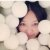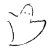KR是首屈一指的知识表示和推理国际会议。与一般AI会议相比，KR会议系列为研究人员研究由推理算法操纵的知识的显式表示提供了更为私密的环境，这为从事人工智能，计算机科学和软件工程的大量工作提供了重要基础。会议强调了知识表示和推理的理论原理以及这些原理及其在工作系统中的实施方式之间的关系。 官网地址：http://dblp.uni-trier.de/db/conf/kr/0+0+0+0+0+0+0+0+0+0+0+0+0+0+0+0+0+0+0+0+0+0+0+0+0+0+0+0+0+0+0+0+0+0+0+0+0+0+0+0+0+0+0+0+0+
Top